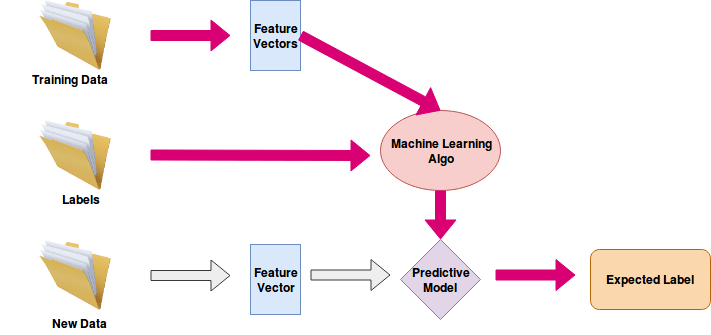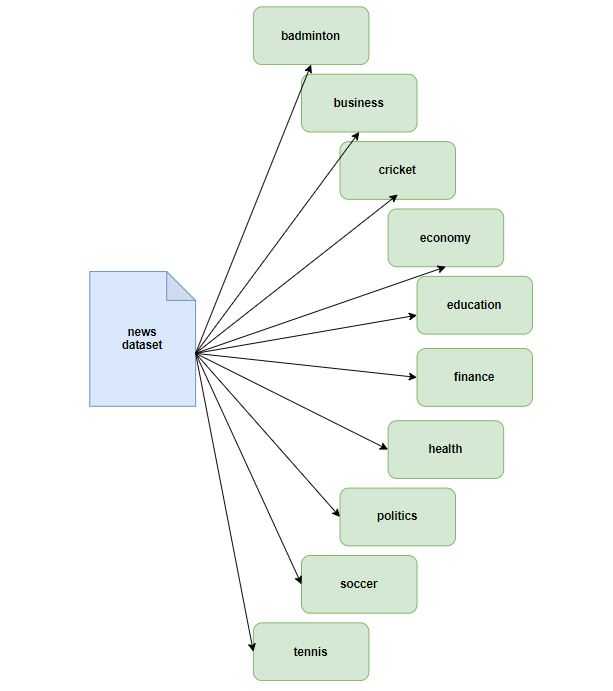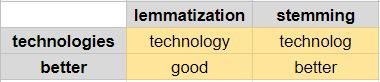Machine Learning, Deep LearningArtificial Intelligence are the popular buzzwords in present trends.

Artificial Intelligence(AI) is the branch of computer science which deals with developing intelligence artificially to the machines which are able to think, act and behave like humans.

Machine Learning(ML) is a subset of AI and is the way to implement artificial intelligence. It is the statistical approach where each instance in a data-set is described by a set of features or attributes. Feature Extraction is key in ML.

Deep Learning(DL) is the next evolution and subset of ML. It is a method of statistical learning that extracts features or attributes from raw data. DL uses a network of algorithms called artificial neural networks which imitates the function of the human neural networks present in the brain. DL takes the data into a network of layers(Input, Hidden & Output) to extract the features and to learn from the data. Let’s end about the DL here – We will discuss more in the coming blogs.outline

In ML/DL, there are models that fall into different categories like supervised, unsupervised & reinforcement learning. In this tutorial, we will discuss Supervised learning which involves an output label associated with each instance in the data-set.Supervised Learning Model Flow Chart

Text(Document) Classification/Text(Document) Categorization is one of the important and typical tasks in supervised ML. This technique allows machines to understand and then categorize text into known organized groups.

In this post, we will look into the supervised learning technique of how classification on the document dataset can be approached with ML algorithms.

Some of the ML algorithms are:

• Naive Bayes.
• Decision Trees.
• Logistic Regression (Linear Model).
• Support Vector Machines (SVM).
• Random Forest.
• K-Means Clustering.
• K-Nearest Neighbour.
• Gaussian Mixture Model.
• Hidden Markov Model. et cetera

Among these ML Algorithms, we will discuss how the Naive Bayes, Logistic Regression and SVM classifier models perform on the data-set feature vectors.

## Datasetnews dataset

Categorized the data-set with the above 10 categories with each category of 1000 entries with content and label as two columns. I would call this dataset a dataframe(df) hereafter in the following code snippets.dataset

## Data Cleaning

Pre-processing of data will have an impact on the output ie., the accuracy, performance of the model. Some of the data-cleaning steps are as follows

• Removing Stop Words. (NLTK)
• Performing Stemming on the text. (NLTK)
• Removing special characters & extra spaces or keeping only Alpha-Numeric characters in the text.
```# NLTK python module for stemming and stopwords removal
from nltk.stem.snowball import SnowballStemmer
from nltk.corpus import stopwords
import string, re```
```stemmer = SnowballStemmer('english') # stemmer
t = str.maketrans(dict.fromkeys(string.punctuation)) # special char removal```
```def clean_text(text):
## Remove Punctuation
text = text.translate(t)
text = text.split()```
```    ## Remove stop words
stops = set(stopwords.words("english"))
text = [stemmer.stem(w) for w in text if not w in stops]

text = " ".join(text)
text = re.sub(' +',' ', text) # extra consecutive space removal
return text

df["content"] = df["content"].apply(clean_text)
```

This data cleaning part is optional – one can test the model accuracy with and without the data cleaning. Removing stop words and performing stemming can take away the context essence from the data.

Stemming removes or stems the last few characters of a word, often leading to meaningless words. Lemmatization considers the context and converts the word to its meaningful base form, which is called Lemma.lemmatization Vs Stemming

We have performed the stemming and stop word removal on the df before the data transformation process.

## Data Transformation

Transforming the data into feature vectors with the following methods

• Count Vectorization.
• TF-IDF Word Vectorization.
• TF-IDF N-Gram Vectorization.

We recommend you go through these feature extraction methods which are explained in detail in one of our blogs.

Dataset(df) has been split into train and validate samples in the range of 75 and 25% respectively by sklearn’s train_test_split function.

Code Snippet to transform data into vectors using the scikit-learn(sklearn) module.

```from sklearn import model_selection, preprocessing
from sklearn.feature_extraction.text import TfidfVectorizer, CountVectorizer```
`'''Assume df is the dataset with columns "content" and "label"'''`
```# split the data into training and validation
train_x, valid_x, train_y, valid_y = model_selection.train_test_split(df['content'], df['label'])```
```# label encode the target variable
encoder = preprocessing.LabelEncoder()
train_y = encoder.fit_transform(train_y)
valid_y = encoder.fit_transform(valid_y)```
```# count vectorization
count_vect = CountVectorizer(analyzer='word', token_pattern=r'\w{1,}')
count_vect.fit(df['content'])
xtrain_count = count_vect.transform(train_x)
xvalid_count = count_vect.transform(valid_x)```
```# word level tf-idf vectorization
tfidf_vect = TfidfVectorizer(analyzer='word', token_pattern=r'\w{1,}', max_features=5000)
tfidf_vect.fit(df['content'])
xtrain_tfidf = tfidf_vect.transform(train_x)
xvalid_tfidf = tfidf_vect.transform(valid_x)```
```# ngram level tf-idf vectorization
tfidf_vect_ngram = TfidfVectorizer(analyzer='word', token_pattern=r'\w{1,}', ngram_range=(2,3), max_features=5000)
tfidf_vect_ngram.fit(df['content'])
xtrain_tfidf_ngram = tfidf_vect_ngram.transform(train_x)
xvalid_tfidf_ngram = tfidf_vect_ngram.transform(valid_x)```

## Training with Naive Bayes, Logistic Regression, and SVM

We use Naive Bayes, Logistic Regression, and SVM algorithms to train the data-set feature vectors to form classifier models that are used for prediction.

From the above data transformation snippet, we have respective train and validation vectorization objects along with their label encoder values. We will use them here to fit the respective classifier and predict the model using the validation sample dataset.

This report_generation function is the common function used by the three ML Algorithms to predict validation data and pass the model’s accuracy.

```from sklearn import linear_model, naive_bayes, svm, metrics
from sklearn.metrics import classification_report

target_names = list(encoder.classes_) # output labels for report generation
```
```def report_generation(classifier, train_data, valid_data, train_y, valid_y):
classifier.fit(train_data, train_y)
predictions = classifier.predict(valid_data)
print("Accuracy :", metrics.accuracy_score(predictions, valid_y))
report = classification_report(valid_y, predictions, output_dict=True, target_names=target_names)
return report```

### Naive Bayes

```# Naive Bayes
classifier = naive_bayes.MultinomialNB()
report = report_generation(classifier, xtrain_count, xvalid_count, train_y, valid_y)
print("NB Count Vectorizer Report :", report['weighted avg'])

# Results
Accuracy : 0.9436
NB Count Vectorizer Report : {'precision': 0.9448178637882411, 'recall': 0.9436, 'f1-score': 0.9434664656369504, 'support': 2500}

report = report_generation(classifier, xtrain_tfidf, xvalid_tfidf, train_y, valid_y)
print("NB TFIDF-Word Report :", report['weighted avg'])

# Results
Accuracy : 0.9416
NB Count Vectorizer Report : {'precision': 0.9430346010709252, 'recall': 0.9416, 'f1-score': 0.9416037073783431, 'support': 2500}```
```report = report_generation(classifier, xtrain_tfidf_ngram, xvalid_tfidf_ngram, train_y, valid_y)
print("NB TFIDF-NGram Report :", report['weighted avg'])

# Results
Accuracy : 0.9208
NB Count Vectorizer Report : {'precision': 0.9233051466162964, 'recall': 0.9208, 'f1-score': 0.9206511260527037, 'support': 2500}```

### Logistic Regression

`# Logistic Regression `
```classifier = linear_model.LogisticRegression()
report = report_generation(classifier, xtrain_count, xvalid_count, train_y, valid_y)
print("LogisticRegression Count Vectorizer Report :", report['weighted avg'])

# Results
Accuracy : 0.9804
LogisticRegression Count Vectorizer Report : {'precision': 0.9806682334322502, 'recall': 0.9804, 'f1-score': 0.9804527264151257, 'support': 2500}

report = report_generation(classifier, xtrain_tfidf, xvalid_tfidf, train_y, valid_y)
print("LogisticRegression TFIDF-Word Report :", report['weighted avg'])

# Results
Accuracy : 0.9792
LogisticRegression TFIDF-Word Report : {'precision': 0.9794911617869886, 'recall': 0.9792, 'f1-score': 0.9792657461379974, 'support': 2500}```
```report = report_generation(classifier, xtrain_tfidf_ngram, xvalid_tfidf_ngram, train_y, valid_y)
print("LogisticRegression TFIDF-NGram Report :", report['weighted avg'])

# Results
Accuracy : 0.932
LogisticRegression TFIDF-NGram Report : {'precision': 0.9329064009056843, 'recall': 0.932, 'f1-score': 0.9320786137751711, 'support': 2500}```

### SVM

```# Support Vector Machines

classifier = svm.SVC(gamma="scale")
report = report_generation(classifier, xtrain_count, xvalid_count, train_y, valid_y)
print("SVM Count Vectorizer Report :", report['weighted avg'])

# Results
Accuracy : 0.9668
SVM Count Vectorizer Report : {'precision': 0.9687847838287942, 'recall': 0.9668, 'f1-score': 0.9672306318670637, 'support': 2500}```
```report = report_generation(classifier, xtrain_tfidf, xvalid_tfidf, train_y, valid_y)
print("SVM TFIDF-Word Report :", report['weighted avg'])

# Results
Accuracy : 0.9804
SVM TFIDF-Word Report : {'precision': 0.980766234757573, 'recall': 0.9804, 'f1-score': 0.9804795388691244, 'support': 2500}```
```report = report_generation(classifier, xtrain_tfidf_ngram, xvalid_tfidf_ngram, train_y, valid_y)
print("SVM TFIDF-NGram Report :", report['weighted avg'])

# Results
Accuracy : 0.9304
SVM TFIDF-NGram Report : {'precision': 0.9324797933370057, 'recall': 0.9304, 'f1-score': 0.9306949638900389, 'support': 2500}```

## conclusion

SVM with TF-IDF Word Vectorizer and Logistic Regression with Count Vectorizer gives better accuracy compared with other ML algorithms tested.

We recommend you train the model without the data cleaning part to check which ML algorithm works better following the similar approach shown above.

Disclaimer: We can not say which model is best here – It all depends on your data. Then, how do we conclude which ML algorithm suits our data?

Selecting the best model for your ML problem is definitely a difficult task. There is an awesome python library called Lazy Predict which helps to understand which models work better for your data without any parameter tuning. Check out the documentation here. In the coming posts, we will discuss the Lazy Predict python module with some examples.